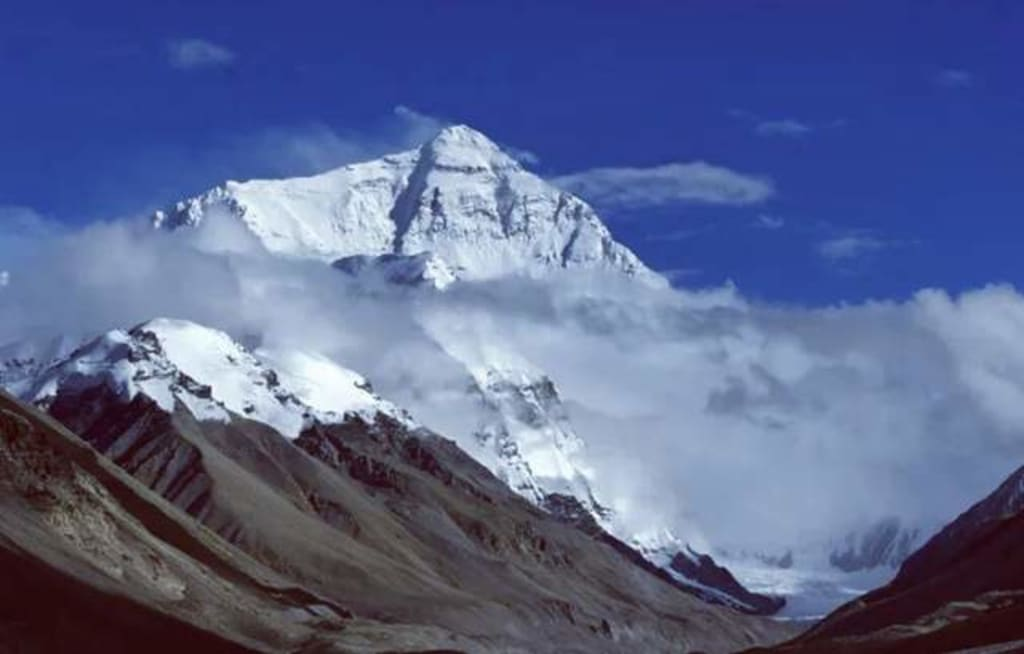# The Earth has high mountains and deep oceans, so why do scientists still say that the Earth is a near-perfect sphere?

## Is the Earth a near-perfect sphere?

By SalolahertyPublished 8 months ago 5 min read
1That the Earth is round is a scientific truism known to modern humans. So how round is the Earth? The answer given by scientists is: The Earth is very round and can be described as a nearly perfect sphere.

This is bound to raise some suspicions because the surface of the Earth has a lot of undulations. At the highest level, the Earth has mountains over 8,000 meters, and at a lower level, the Earth has trenches over 10,000 meters deep. If this is the case, why do scientists still say that the Earth is round?

Wrong view.

There is a view that the reason why the Earth appears to be round should be the effect of the Earth's oceans. Simply put, the large amount of seawater "fills in" the undulations on the Earth's surface. If all the seawater on the Earth's surface was pumped away, the Earth would not look round. The following oft-quoted image seems to validate this idea.

Is this the case? The answer is no because this picture is a very exaggerated depiction of the difference in height of the "geoid" (i.e., the gravitational equipotential plane that coincides with sea level at rest), not "what the Earth would look like if all the water were pumped away.

If you look closely, you will see that the scale on the right side of the graph is plus or minus 80, which is measured in "meters", or "m". Compared to the radius of the Earth, which is about 6371 kilometers, the difference in the height of the "geodetic level" is a few orders of magnitude, which can be said to be very small. InToxpress the nuances of the "geodetic level" more visually, scientists have chosen to exaggerate the way to express it.

Scientists say that the Earth is round, but it is an objective description of the Earth.

The Earth has trenches over 10,000 meters deep and mountains 8,000 meters high. This is indeed true. Known data show that Mount Everest is the highest mountain on Earth, with an altitude of about 8,848 meters. The deepest trench on Earth is the Marianas Trench, which is 11,034 meters deep. This is indeed very considerable for human beings, but compared with the volume of the Earth, it is not worth mentioning.

The average radius of the Earth is about 6371 kilometers. A simple calculation shows that the height of the highest mountain on Earth is only about 0.14% of the Earth's radius, while the depth of the deepest trench on Earth is about 0.17% of the Earth's diameter. What is this concept?

Let's put it this way, the radius of a standard basketball is about 123mm. that is, if we shrink the earth to the size of a standard basketball, the highest bump on the earth's surface is about 0.17mm, the lowest depression is about 0.2mm, the height difference is only 0.37mm, shallower than the depth of the grain on the basketball, can be said to be very flat.

Of course, the flatness of the surface does not mean that the Earth is very round, what determines the roundness of the Earth is the overall roundness error of the Earth.

The Earth is always rotating, and in the process, will produce "centrifugal force". This force is an imaginary force, the size of which is proportional to the angular velocity. On the surface of the Earth, the lower the latitude, the greater the angular velocity, and the greater the "centrifugal force". This causes a certain degree of bulging at the Earth's equator so that the Earth's polar radius is greater than the Earth's equatorial radius.

In the past, scientists have measured various data on the Earth through artificial satellites. The measured data show that the polar radius of the Earth is about 6356.8 km and the equatorial radius is about 6378.1 km, a difference of 21.3 km. Accordingly, the overall circularity error of the Earth is "21.3/(6356.8+6378.1)/2", which is calculated as

In contrast, for a competition diameter of 40mm for high-quality table tennis, the roundness error should be controlled within 0.1mm, that is, 5 ‰ (five thousandths), that is, if we reduce the diameter of the earth to 40mm, the roundness error is fully consistent with the standards of a high quality table tennis. As for how to round a high-quality ping pong ball, I believe you should have an idea.

From the above data analysis, we can see that the earth is indeed very round. The actual images of the Earth taken by us humans through various spacecraft are also the same. From space, the Earth always appears in front of us as a nearly perfect sphere.

What makes the Earth's shape so round?

The answer is gravity. Of the four fundamental forces known in the universe, gravity is the weakest. Because gravity is a long-range force, there is only "gravitational force" and no "repulsive force", so gravity can be infinitely superimposed.

We know that the magnitude of gravity is proportional to the mass, so when the mass of a celestial body reaches a certain level, its gravitational force can be superimposed enough to make the matter that constitutes the body behave as fluid on a macroscopic scale.

As long as the mass of a celestial body is large enough, even if the body is composed mainly of rocky material, in this case, the body will naturally evolve into a sphere-like shape when the material constituting the body reaches hydrostatic equilibrium.

Science
1

### Salolaherty

Science is like a tree， how also can not hold one

How does it work?

There are no comments for this story

Be the first to respond and start the conversation.